# [分享]单层钢结构厂房--基础设计1．地基承载力特征值和基础材料

±0.000m~－0.6m，回填土含腐殖质，γ=16KN/m3fak=80KN/m2E=300N/mm2

0.6m~－2.70m，一般亚粘土，γ=20KN/m3fak=230KN/m2E=500N/mm2

2.70m以下为风化混合土， fak=300KN/m2E=600~1000N/mm2

fa=fak+ηbγ(b－3)+ηdγm(d－0.5)

=230+1.6×[(16×0.6+20×0.4)/1.0]×(1.0－0.5)

=244.1KN/m2

2． 基础底面内力及基础底面积计算

N=102.82KN，V=32.21KN，M=0

Nk=81.18KN，Vk=25.06KN，Mk=0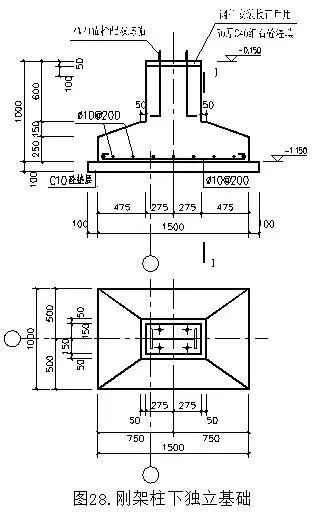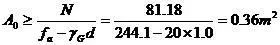(1.1~1.4)A0估计偏心受压基础的底面积A：

A=(1.1~1.4)×0.36=0.40~0.50m2

A=bl=1.5×1.0m=1.5m2W=0.375m3，基础的形状、尺寸及布置如图。

Gk=24×(1.5×1.0×0.4)+16×(1.5×1.0×0.6)

=28.80KN

Nk=81.18+28.80=109.98KN

Mk=25.06×1.0=25.06KN·m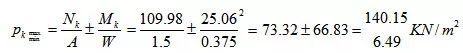1.2fa=292.92KN/m2>pkmaxpkmin>0，(pkmax+pkmin)/2<fa

#### 3． 验算基础变阶处的受冲切承载力

N=102.82KN，M=32.21×1.0=32.21KN·m，e=M/N=0.313m

N合力作用点至基础底面最大压力边缘的距离a=1.5/2－0.313=0.437m

pnmax=2N/(3la)=2×102.82/(3×1.0×0.437)=156.86KN/m2

pn=100.03KN/m2h0=400－45=355mm，at=300mm

ab=300+355×2=1010mm>L，取ab=1000mm，am=(at+ab)/2=650mm

Fu=0.7βhpftamh0=0.7×1.0×1.1×650×355=177.7×103N=177.7KN

Fl=pjAl=156.86×[(1.5/2－0.55/2－0.355)×1.0]=74.5KN<Fu，满足要求。

#### 4． 基础底面配筋计算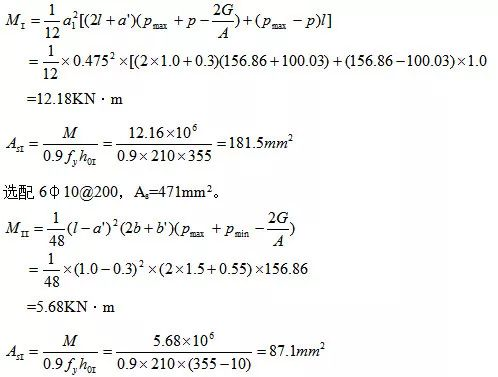#### 2．荷载计算

50mm厚玻璃棉板0.05KN/m2

0.35×(7.2－1.2)=2.10 KN/m

240mm厚浆砌普通砖0.24×18=4.32 KN/m2

18mm厚水泥砂浆粉刷内外墙面0.018×20×2=0.72 KN/m2

#### 3．基础底面宽度的确定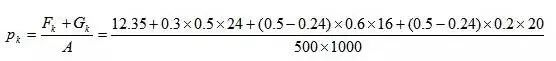=39.0KN/m2<<fa=244.1 KN/m2，满足要求。

4． 验算砖墙底部截面处的承载力

H0=2H=4.0m，μ1=1.2，μ2=1.0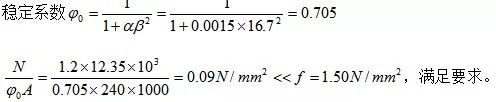发表于2020-05-18   |  只看该作者

2

回帖后跳转到最后一页

### 最新帖子